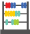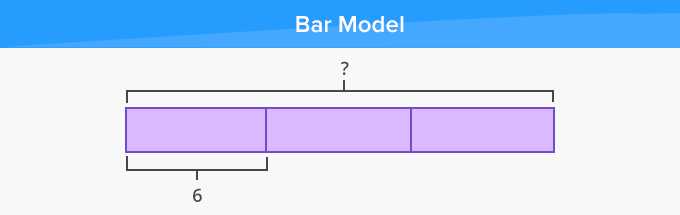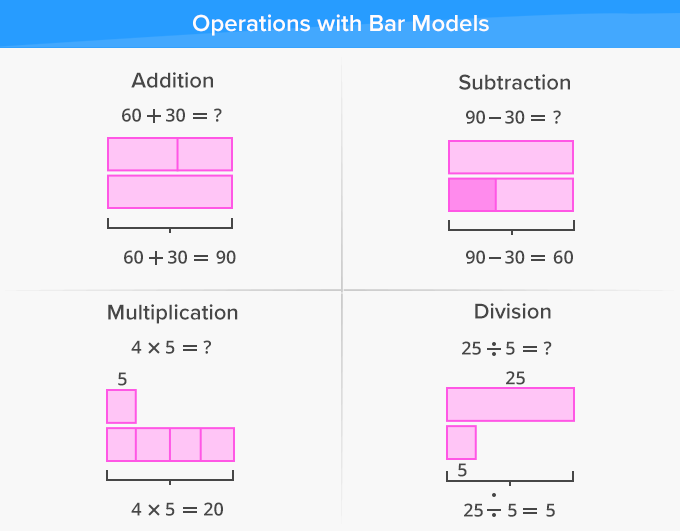# Bar Model - Definition with Examples

The Complete K-5 Math Learning Program Built for Your Child

• 40 Million Kids

Loved by kids and parent worldwide

• 50,000 Schools

Trusted by teachers across schools

• Comprehensive Curriculum

Aligned to Common Core

##Let's learn!

What is a bar model?
In math, a bar model can be defined as a pictorial representation of a number in the form of bars or boxes used to solve number problems. Bar models help us to attain an understanding of how a problem needs to be solved and calculated.

Here, for instance, one rectangle or box of the bar model represents a value of 6. We have to find 3 times the value of one bar.Bar models help us to understand what operations, whether addition, subtraction, multiplication or/and division should be used to solve a word problem.

Here’s how we can represent different operations using bar models.Fun Facts Fraction bars or strips  are also a type of bar models that represent fractions.

##Let's do it!

While solving math word problems in worksheets, ask your 2nd grade child to draw bar models to understand which operations should be used and how the problem should be solved. Model to build understanding of bar models.

##Related math vocabulary

Won Numerous Awards & Honors